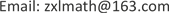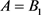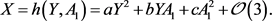﻿ 具有饱和发生率和饱和治疗函数的离散SIS传染病模型的分岔分析 Bifurcation Analysis of Discrete SIS Model with Saturated Incidence and Saturated Treatment Function

Dynamical Systems and Control
Vol. 08  No. 04 ( 2019 ), Article ID: 32179 , 6 pages
10.12677/DSC.2019.84026

Bifurcation Analysis of Discrete SIS Model with Saturated Incidence and Saturated Treatment Function

Yuqing Chen, Mingxuan Liang, Qiaomei Tang, Xiaoliang Zhou

School of Mathematics and Statistics, Lingnan Normal University, Zhanjiang GuangdongReceived: Aug. 23rd, 2019; accepted: Sep. 1st, 2019; published: Sep. 17th, 2019ABSTRACT

In this paper, we discuss the discrete SIS models with saturation incidence and saturation treatment function. We obtain the type and hyperbolicity of the disease-free equilibrium point. We also use the central manifold theorem and the bifurcation theory to obtain the conditions of Transcritical bifurcation and flip bifurcation for disease-free equilibrium point. Finally, the biological explanation of bifurcation is given.

Keywords:Discrete SIS Model, Stability, Transcritical Bifurcation, Flip Bifurcation1. 引言

$\left\{\begin{array}{l}\frac{\text{d}S}{\text{d}t}=A-dS-\frac{\lambda SI}{1+kI}+vI+\frac{rI}{1+\alpha I},\\ \frac{\text{d}I}{\text{d}t}=\frac{\lambda SI}{1+kI}-\left(d+v+u\right)I-\frac{rI}{1+\alpha I},\end{array}$ (1)

$\left\{\begin{array}{l}{S}_{n+1}=\left(1-d\right){S}_{n}-\lambda {I}_{n}\left({S}_{n}+\frac{A}{d}\right)+k\lambda {I}_{n}^{2}\left({S}_{n}+\frac{A}{d}\right)+v{I}_{n}+r{I}_{n}-\alpha r{I}_{n}^{2},\\ {I}_{n+1}=\lambda {I}_{n}\left({S}_{n}+\frac{A}{d}\right)-k\lambda {I}_{n}^{2}\left({S}_{n}+\frac{A}{d}\right)-\left(d+u+v-1\right){I}_{n}-r{I}_{n}+\alpha r{I}_{n}^{2},\end{array}$ (2)

${S}_{n}$${I}_{n}$ 指的是n时刻易感者和染病者的数量，假定 $S\left(0\right)>0,I\left(0\right)>0$ ，系数参数 $A,d,u,v,\lambda ,r$ 都是正的， $k,\alpha$ 非负。

2. 平衡点分析

$DF\left({E}_{0}\right)=\left[\begin{array}{cc}1-d& -\frac{\lambda A}{d}+v+r\\ 0& \text{​}\frac{\lambda A}{d}-\left(d+u+v+r-1\right)\end{array}\right],$

${\lambda }_{1}=\left(1-d\right),{\lambda }_{2}=\frac{\lambda A}{d}-\left(d+u+v+r-1\right).$

${B}_{1}=\frac{d\left(d+u+v+r\right)}{\lambda },{B}_{2}=\frac{d\left(d+u+v+r-2\right)}{\lambda }.$

i) 当$A={B}_{2}$ 时， ${E}_{0}$ 非双曲；

ii) 当 ${B}_{1} 时， ${E}_{0}$ 全局渐近稳定；当 $A>{B}_{2}$$A<{B}_{1}$ 时， ${E}_{0}$ 是鞍点。

3. 跨临界分岔和Flip分岔

${M}_{1}={d}^{2},{M}_{2}=-d\left(d+u\right),{M}_{3}=d\left(d-2\right),{M}_{4}=d\left(2-d-u\right),$

$\omega =\frac{d\left(v+r\right)-\lambda A}{\lambda A-d\left(u+v+r\right)},{\omega }_{1}=\frac{{M}_{2}-\lambda {A}_{1}}{\lambda {A}_{1}-{M}_{1}},{\omega }_{2}=\frac{{M}_{4}-\lambda {A}_{2}}{\lambda {A}_{2}-{M}_{3}}.$

$D{F}_{A}\left({E}_{0}\right)=\left[\begin{array}{cc}1-d& -\frac{\lambda A}{d}+v+r\\ 0& \text{​}\frac{\lambda A}{d}-\left(d+u+v+r-1\right)\end{array}\right].$

${\left(1,0\right)}^{\tau },{\left(\omega ,1\right)}^{\tau },$ (3)

$\left[\begin{array}{c}S\\ I\end{array}\right]=\left[\begin{array}{cc}1& \omega \\ 0& 1\end{array}\right]\left[\begin{array}{c}X\\ Y\end{array}\right],$ (4)

$\left[\begin{array}{c}X\\ Y\\ A\end{array}\right]↦\left[\begin{array}{ccc}1-d& 0& 0\\ 0& 1& 0\\ 0& 0& 1\end{array}\right]\left[\begin{array}{c}X\\ Y\\ A\end{array}\right]+\left[\begin{array}{c}-\left(1+\omega \right)\left[\lambda Y\left(Xu+\omega Y\right)-k\lambda {Y}^{2}\left(X+\omega Y+\frac{A}{d}\right)+\alpha r{Y}^{2}\right]\\ \lambda Y\left(X+\omega Y\right)-k\lambda {Y}^{2}\left(X+\omega Y+\frac{A}{d}\right)+\alpha r{Y}^{2}\\ 0\end{array}\right].$ (5)时，有 ${\lambda }_{2}=1,{\lambda }_{1}=1-d$ ，由文献  的定理2.1.4可知，无病平衡点 $\left(X,Y\right)=\left(0,0\right)$ 的稳定性与分岔可通过研究在中心流形上一参数族映射来确定，令 $\xi ={B}_{1},{A}_{1}=A-\xi$ ，在 ${A}_{1}$ 附近的中心流形有如下形式：

${W}_{loc}\left(0,0\right)=\left\{\left(X,Y,{A}_{1}\right)\in {R}^{3}|X=h\left(Y,{A}_{1}\right),h\left(0,0\right)=0,Dh\left(0,0\right)=0,|Y|<\delta ,|{A}_{1}|<\delta \right\}.$$\begin{array}{l}\mathcal{N}\left(h\left(Y,{A}_{1}\right)\right)=h\left(Y,{A}_{1}\right)-\left(1-d\right)h\left(Y,{A}_{1}\right)\\ \text{\hspace{0.17em}}\text{\hspace{0.17em}}+\left\{\left(1+\omega \right)\lambda Y\left[h\left(Y,{A}_{1}\right)+\omega Y\right]-k\lambda {Y}^{2}\left[h\left(X,{A}_{1}\right)+\omega Y+\frac{A}{d}\right]+\alpha r{Y}^{2}\right\}\\ =0.\end{array}$

$a=\frac{\left({M}_{1}+{M}_{2}\right)\left[-\lambda d{M}_{2}+k\lambda \xi {M}_{1}-\alpha rd{M}_{1}\right]}{{\left(d{M}_{1}\right)}^{2}},b=c=0,$

$X=h\left(Y,{A}_{1}\right)=a{Y}^{2}+\mathcal{O}\left(3\right).$

$Y↦{\Phi }_{{A}_{1}}\left(Y\right)=Y+\left[\lambda Y\left(a{Y}^{2}+{\omega }_{1}Y\right)-k\lambda {Y}^{2}\left(a{Y}^{2}+{\omega }_{1}Y+\frac{{A}_{1}+\xi }{d}\right)+\alpha r{Y}^{2}\right]+\mathcal{O}\left(5\right).$

$A={B}_{1}$ 时，验算如下式子成立：

$\frac{\partial {\Phi }_{{A}_{1}}}{\partial {A}_{1}}\left(0,0\right)=0,\frac{\partial {\Phi }_{{A}_{1}}}{\partial Y\partial {A}_{1}}\left(0,0\right)\ne 0,\frac{{\partial }^{2}\Phi }{\partial {Y}^{2}}\left(0,0\right)\ne 0.$ (6)

$Y↦{\Phi }_{{A}_{1}}\left(Y\right)=Y+\left[\lambda Y\left(a{Y}^{2}+{\omega }_{1}Y\right)-k\lambda {Y}^{2}\left(a{Y}^{2}+{\omega }_{1}Y+\frac{{A}_{1}+\xi }{d}\right)+\alpha r{Y}^{2}\right]$ (7)

$X=h\left(Y,{A}_{2}\right)={a}^{*}{Y}^{2}+\mathcal{O}\left(3\right).$

${a}^{*}=\frac{\left({M}_{3}+{M}_{4}\right)\left[\lambda d{M}_{4}+k\lambda \zeta {M}_{3}-\alpha rd{M}_{3}\right]}{{\left(d{M}_{3}\right)}^{2}}$

$Y↦{\Phi }_{{A}_{2}}\left(Y\right)=-Y+\left[\lambda Y\left({a}^{*}{Y}^{2}+{\omega }_{2}Y\right)-k\lambda {Y}^{2}\left({a}^{*}{Y}^{2}+{\omega }_{2}Y+\frac{{A}_{2}+\zeta }{d}\right)+\alpha r{Y}^{2}\right]+\mathcal{O}\left(5\right).$

$A=\frac{d\left(d+u+v+r-2\right)}{\lambda }$ 时，有 ${\lambda }_{2}=-1,{\lambda }_{1}=1-d$ ，系统(2)在无病平衡点${\alpha }_{1}=-\frac{2{\lambda }^{2}\left({M}_{3}+{M}_{4}\right)}{{M}_{3}^{2}}-\frac{2k\lambda }{d},{\alpha }_{2}=\frac{\lambda {M}_{3}}{{M}_{4}}\left(1-2k\right)-\frac{\lambda k\zeta }{d}+2\lambda {a}^{*}+\alpha r.$

4. 分岔生物学解释

Bifurcation Analysis of Discrete SIS Model with Saturated Incidence and Saturated Treatment Function[J]. 动力系统与控制, 2019, 08(04): 242-247. https://doi.org/10.12677/DSC.2019.84026

1. 1. 马知恩, 周义仓, 吴建宏. 传染病建模与动力学[M]. 北京: 高等教育出版社, 2009.

2. 2. Safi, M.A., et al. (2013) Qualitative Analysis of an Age-Structured SEIR Epidemic Model with Treatment. Applied Mathematics & Computation, 219, 10627-10642.
https://doi.org/10.1016/j.amc.2013.03.126

3. 3. Wang, W. and Ruan, S. (2015) Bifurcations in an Epidemic Model with Constant Removal Rate of the Infectives. Journal of Mathematical Analysis & Applications, 291, 775-793.
https://doi.org/10.1016/j.jmaa.2003.11.043

4. 4. Allen, L.J.S. (1994) Some Discrete-Time SI, SIR, and SIS Epidemic Models. Mathematical Biosciences, 124, 83-105.
https://doi.org/10.1016/0025-5564(94)90025-6

5. 5. Castillo-Chavez, C. and Yakubu, A.A. (2015) Discrete-Time SIS Models with Complex Dynamics. Nonlinear Analysis, 47, 4753-4762.
https://doi.org/10.1016/S0362-546X(01)00587-9

6. 6. Zhang, X. and Liu, X. (2008) Backward Bifurcation of an Epidemic Model with Saturated Treatment Function. Journal of Mathematical Analysis & Applications, 348, 433-443.
https://doi.org/10.1016/j.jmaa.2008.07.042

7. 7. Liu, W.M., Levin, S.A. and Iwasa, Y. (1986) Influence of Nonlinear Incidence Rates upon the Behavior of SIRS Epidemiological Models. Journal of Mathematical Biology, 23, 187-204.
https://doi.org/10.1007/BF00276956

8. 8. Zhou, T., Zhang, W. and Lu, Q. (2014) Bifurcation Analysis of an SIS Epidemic Model with Saturated Incidence Rate and Saturated Treatment Function. Journal of Dynamics & Control, 226, 288-305.
https://doi.org/10.1016/j.amc.2013.10.020

9. 9. Wiggins, S. (1990) Introduction to Applied Nonlinear Dynamical Systems and Chaos. 2nd Edition, Springer-Verlag, New York.
https://doi.org/10.1007/978-1-4757-4067-7

10. 10. Guckenheimer, J. and Holmes, P.J. (1983) Nonlinear Oscillations, Dynamical Systems and Bifurcations of Vector Fields. Springer-Verlag, New York.
https://doi.org/10.1007/978-1-4612-1140-2